## 1 Introduction

This tutorial provides an introduction to algebraic program analysis, focusing upon techniques for (numerical) invariant generation and termination analysis. By reading this paper, you will learn the answers to the following questions:

• How does one design an algebraic program analysis?

• What new opportunities does algebraic program analysis enable?

• What are the limitations and important open problems in algebraic program analysis?

The origin of algebraic program analysis is the algebraic approach to solving path problems in graphs [1, 6, 48, 59]: (1) compute a regular expression recognizing a set of paths of interest, and (2) interpret that regular expression within an algebraic structure corresponding to the problem at hand. Various path problems (e.g., computing shortest paths, path-finding problems, and dataflow analysis) can be solved by using different algebraic structures to interpret regular expressions.

In the context of program analysis, the graph of interest is a control flow graph for a program, and the algebra defines a space of summaries (approximations of program behavior) and a means for composing them. The algebraic approach amounts to computing a summary for a program in “bottom-up” fashion, building summaries for larger and larger subprograms by applying the operators of the summary algebra.

The general pattern of an algebraic program analysis is: given a system of (recursive) equations defining the semantics of a program, (1) symbolically compute a closed-form solution, and then (2) interpret the closed form within an algebraic structure corresponding to the analysis. The algebraic approach can be contrasted with classical iterative abstract interpretation, which also starts with a system of (recursive) equations defining the semantics of a program. However, the iterative approach is to (a) interpret the operations in the equations in an abstract domain, and then (b) solve the equations over the abstract domain by successive approximation. Thus, the classical approach is one of “interpret and then solve,” whereas the algebraic approach is “solve and then interpret.”

The algebraic approach can be applied to various kinds of equations and algebraic structures. Three cases we consider in this article, and the corresponding kind of program-analysis problems they can be used to solve, are:

• Section 2 (Non-recursive) program summarization: left-linear equations over regular algebras.

• Section 4 Linearly-recursive procedure summarization: linear equations over tensor-product domains.

• Section 5 Conditional termination analysis: right-linear equations over $$\omega$$-regular algebras.

Why Algebraic Program Analysis? Algebraic program analysis is a general framework for understanding compositional program analyses. The principle of compositionality states that “the meaning of a complex expression is determined by its structure and the meanings of its constituents” . A program analysis is compositional when the result of analyzing a composite program is a function of the results of analyzing its components. Compositionality enables program analyses to scale to large programs, to be parallelized, to be applied incrementally, and to be applied to incomplete programs . Algebraic program analysis provides a structure in which to think about how to design such an analysis.

Insistence upon compositionality also demands a different perspective on program analysis, which can suggest solutions to problems that may otherwise not be apparent. We demonstrate this principle with a series of examples that illustrate a variety of different ideas that are enabled by thinking of program analysis in compositional terms.

Last, the algebraic framework enables a style of reasoning about the behavior of program analyses themselves. By exploiting compositionality, it is possible to design effective algebraic analyses that satisfy certain laws (e.g., monotonicity—“more information in yields more information out”). Analyses can be classified on the basis of algebraic laws that they satisfy, and we can reason how program transformation affects analysis using these laws.

Why Not Algebraic Program Analysis? While compositionality brings many desirable properties, it comes at the price of losing context. Compositionality requires that the analysis of a program component is a function of the source code of that component, and therefore cannot depend on the surrounding context in which the component appears in the program. Many program analysis techniques make essential use of context, for example:

• In an iterative abstract interpreter, which propagates information about reachable states from the program entry forwards, the analysis of a component depends on every component that may precede it in an execution.

• In a refinement-based software model checker, which inspects paths that go from entry to an error state, the analysis of a component depends on the whole program.

One of the main challenges of designing a good algebraic program analysis is to overcome this loss of contextual information.

Secondly, algebraic program analysis is less general than iterative program analysis, in the sense that any set of semantic (in)equations can be solved iteratively using the same basic algorithm, whereas each particular type of equation system requires a specialized algorithm. Some problems—e.g., resolving semantic equations of recursive procedures—have no known practical algebraic solutions.

## 2 Regular Algebraic Program Analysis

This section describes the algebraic approach to solving path problems in graphs [1, 6, 48, 59]. The basic structure of the method is to use regular expressions to capture the set of paths of a graph, and then interpret these expressions to obtain a desired result. We illustrate the approach by considering the problem of computing shortest paths, and then show how it can be applied to numerical invariant generation.

First, we establish some basic definitions. The syntax of regular expressions over an alphabet $$\varSigma$$ is as follows: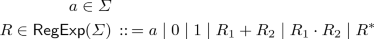We will sometimes use juxtaposition $$R_1R_2$$ (rather than $$R_1\cdot R_2$$) to denote concatenation.

The semantics of regular expressions over $$\varSigma$$ is given by a $$\varSigma$$-interpretation $$\mathscr {I} = \left\langle \mathbf {A},f \right\rangle$$, which consists of regular algebra $$\mathbf {A}$$ and a semantic function f. A regular algebra $$\mathbf {A} = \left\langle A,0^A,1^A,+^A,\cdot ^A,^{*^A} \right\rangle$$ is an algebraic structure consisting of a set A (called its universe) equipped with two distinguished elements $$0^A,1^A \in A$$, two binary operations $$+^A$$ (choice) and $$\cdot ^A$$ (sequencing), and a unary operation $$(-)^{*^A}$$ (iteration).Footnote 1 When the algebra is clear from context, we will drop the superscript. A semantic function $$f: \varSigma \rightarrow A$$ maps each letter in $$\varSigma$$ to an element of $$\mathbf {A}$$’s universe.

A $$\varSigma$$-interpretation $$\mathscr {I} = \left\langle \mathbf {A},f \right\rangle$$ assigns to each regular expression R over $$\varSigma$$ to an element $$\mathscr {I}\llbracket {R}\rrbracket$$ of $$\mathbf {A}$$ by interpreting each letter according to the semantic function and each regular operator using its counterpart in $$\mathbf {A}$$:

Notice that the interpretation is compositional: for any expression R, $$\mathscr {I}\llbracket {R}\rrbracket$$ is a function of the top-level operator in R and the interpretations of its sub-expressions.

### Example 1

(Standard interpretation). The standard interpretation of regular expressions is the language interpretation, $$\mathscr {L} = \left\langle \mathbf {L},\ell \right\rangle$$ where $$\mathbf {L}$$ is the regular algebra of languages. The universe of the interpretation is the set of regular languages over $$\varSigma$$, $$0 \triangleq \emptyset$$ is the empty language, $$1 \triangleq \left\{ \epsilon \right\}$$ is the singleton language containing the empty word, and the operators are

\begin{aligned} X + Y&\triangleq X \cup Y&\text {Union}\\ X \cdot Y&\triangleq \left\{ xy : x \in X, y \in Y \right\}&\text {Concatenation}\\ X^{*}&\triangleq \left\{ x_1x_2\dots x_n : x_1,\dots ,x_n \in X \right\}&\text {Kleene closure} \end{aligned}

The semantic function $$\ell$$ maps each letter a to the singleton language $$\left\{ a \right\}$$. For any regular expression R, $$\mathscr {L}\llbracket {R}\rrbracket$$ is the (regular) set of words recognized by R.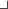We now describe how non-standard interpretations can be used to solve problems over directed graphs. A directed graph $$G = \left\langle V,E \right\rangle$$ consists of a finite set of vertices V and a finite set of directed edges $$E \subseteq V \times V$$. A path in G is a finite sequence $$e_1e_2\dots e_n$$ with $$e_i\in E$$ such that for each i, the destination of $$e_i$$ matches the source of $$e_{i+1}$$. A path expression (in G) is a regular expression over the alphabet of edges E that recognizes a set of paths in G. For any pair of vertices $$u, v \in V$$, there is a path expression $$\textit{PathExp}_{G}(u,v)$$ that recognizes exactly the set of paths in G that begin at u and end at v. There are several ways to compute path expressions. The classical method is Kleene’s algorithm  for computing a regular expression for a finite state automaton (thinking of G as an automaton over the alphabet E with start state u and final state v). For sparse graphs, there are more efficient alternatives to Kleene’s algorithm, in particular Tarjan’s algorithm . The insight of the algebraic approach to path problems is that these algorithms can be re-used for multiple purposes: first use a path expression algorithm to find a regular expression recognizing a set of paths of interest, and then compute a problem-dependent (non-standard) interpretation of that expression.

### Example 2

(Shortest paths). Consider the integer-weighted graph depicted in Fig. 1a. Suppose that we wish to compute the length of the shortest path from a to c. We begin by computing a path expression recognizing all paths from a to c:

$$\left( \left\langle a,b \right\rangle \left\langle b,d \right\rangle \left( \left\langle d,e \right\rangle \left\langle e,d \right\rangle \right) ^*\left\langle d,a \right\rangle \right) ^*\left\langle a,b \right\rangle \left( \left\langle b,c \right\rangle + \left\langle b,d \right\rangle \left( \left\langle d,e \right\rangle \left\langle e,d \right\rangle \right) ^*\left\langle d,c \right\rangle \right)$$

This path expression can be represented succinctly by the directed acyclic graph (DAG) pictured in Fig. 1b. Define the distance interpretation $$\mathscr {D}$$ where the semantic function maps each edge to its weight, and the algebra’s universe consists of the integers along with $$\pm \infty$$, 0 is interpreted as $$\infty$$, 1 as 0, and the operators are as follows:

\begin{aligned} d_1 + d_2&\triangleq \min (d_1,d_2)&\text {Minimum}\\ d_1 \cdot d_2&\triangleq d_1 + d_2&\text {Addition}\\ d^*&\triangleq {\left\{ \begin{array}{ll} -\infty &{} \text {if } d < 0\\ 0 &{} \text {otherwise} \end{array}\right. }&\text {Closure} \end{aligned}

The weight of the shortest weighted path from a to c is $$\mathscr {D}\llbracket {\textit{PathExp}_{G}(a,c)}\rrbracket = 1$$, which can be calculated efficiently by interpreting the path expression DAG “bottom-up” (see gray labels in Fig. 1b).Algebraic path-finding can be used to generate invariants by representing a program by a control flow graph, and interpreting path expressions within an algebra of program summaries. A control flow graph (CFG) $$G = \left\langle V,E,r,C \right\rangle$$ is a directed graph $$\left\langle V,E \right\rangle$$ with a distinguished root (or entry) vertex $$r \in V$$, and where each edge $$e \in E$$ is labeled by a command C(e); see Fig. 2a for an example. In the remainder of this section, we give examples of interpretations that can be used to generate (numerical) program summaries.

### 2.1 Transition-Formula Interpretations

Fix a finite set of variables, X, representing the variables of a program. A transition formula is a logical formula $$F(X,X')$$ whose free variables range over X and a set of “primed copies” $$X' \triangleq \left\{ x' : x \in X \right\}$$. For the purposes of this exposition, we further suppose that variables range over integers, and that transition formulas are expressed in the language of linear integer arithmetic. A transition formula can be interpreted as a binary relation $$\rightarrow _F$$ over states $$\textsf {State}\triangleq \mathbb {Z}^X$$, where $$s \rightarrow _F s'$$ if and only if F is true when s is used to interpret the un-primed variables and $$s'$$ is used to interpret the primed variables. For example, if F is the transition formula

\begin{aligned} F \triangleq x' = x + 1 \wedge y = y' \wedge x < y\ , \end{aligned}

then we have

\begin{aligned} s \rightarrow _F s' \iff s'(x) = s(x) + 1, s(y) = s'(y), \text { and } s(x) < s(y)\ . \end{aligned}

Suppose that $$G = \left\langle V,E,r,C \right\rangle$$ is a control flow graph, where commands range over assignments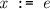and assumptions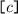, where e is a linear integer term and c is a linear arithmetic formula. (An assumption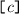is a command that does not change the program state, but which can only be executed if the formulaholds.) We define a semantic function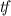that maps each control flow edge into the universe of transition formulas by translating the command associated with the edge into logic: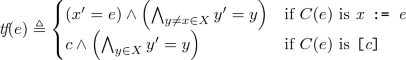We define an algebra of transition formulas as follows:

\begin{aligned} 0&\triangleq \textit{false}&\text {Empty relation}\\ 1&\triangleq \bigwedge _{x \in X} x' = x&\text {Identity relation}\\ F + G&\triangleq F \vee G&\text {Union}\\ F \cdot G&\triangleq \exists X''. F(X,X'') \wedge G(X'', X')&\text {Relational composition}\\ \end{aligned}

Above and elsewhere, we use positional notation for substitution; e.g., $$F(X,X'')$$ denotes the formula obtained by replacing all the $$X'$$ symbols with “double primed” symbols in $$X''$$ (and leaving the un-primed X symbols as they are). Intuitively, $$F^*$$ should be interpreted as the reflective transitive closure of F. However, in general it is not possible to compute the reflexive transitive closure of a formula (nor even to represent it as a formula). Hence, we must be content with an over-approximate transitive closure operator. There are many different methods for over-approximating transitive closure, so we speak of the family of algebras of transition formulas, which have the same basic structure and differ only in the interpretation of the iteration operator. In the remainder of this section, we describe a selection of methods for implementing the iteration operator. Disclaimer: for each example, the presentation differs somewhat (sometimes substantially) from the cited source. The examples should be read as “how the cited analysis might be presented in the algebraic framework.”

### Example 3

(Transitive Predicate Abstraction ). Fix a set of variables X. Say that a transition formula $$p(X,X')$$ is

• reflexive if $$\bigwedge _{x \in X} x = x' \models p(X,X')$$

• transitive if $$p(X,X') \wedge p(X',X'') \models p(X,X'')$$

Let P be a finite set of candidate reflexive and transitive transition formulas. For example we might choose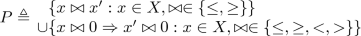We can define an iteration operator that over-approximates the reflexive transitive closure of a formula F by the conjunction of the subset of P that is entailed by F:### Example 4

(Interval analysis ). Let $$F(X,X')$$ be a transition formula. An inductive interval invariant for F assigns to each variable $$x \in X$$ a pair of integers $$a_x,b_x \in \mathbb {Z}$$ such that if s is a state such that $$s(x) \in [a_x,b_x]$$ for all $$x \in X$$ and $$s \rightarrow _F s'$$, then $$s'(x) \in [a_x,b_x]$$ for all $$x \in X$$. Monniaux showed that it is possible to determine optimal inductive interval invariants by posing the inductive-invariance condition symbolically and quantifying over the bounds .

Let $$P = \left\{ p_x : x \in X \right\}$$ and $$Q \triangleq \left\{ q_x : x \in X \right\}$$ be sets of fresh variables, which we use to the lower and upper bounds of intervals, respectively. The set of inductive interval invariants for a formula F can be represented by the formula

\begin{aligned} \textit{Inv}(F,P,Q) \triangleq \forall X,X'. \left( F \wedge \bigwedge _{x \in X} p_x \le x \le q_x\right) \Rightarrow \left( \bigwedge _{x \in X} p_x \le x' \le q_x \right) \end{aligned}

That is, the models of $$\textit{Inv}$$ (which assign integers to the lower and upper bound variables P and Q) are in one-to-one correspondence with the interval invariants of F. We may universally quantify over all inductive interval invariants to arrive at the following iteration operator:

$$F^* \triangleq \forall P,Q. \left( \textit{Inv}(F,P,Q) \wedge \bigwedge _{x \in X} p_x \le x \le q_x\right) \Rightarrow \left( \bigwedge _{x \in X} p_x \le x' \le q_x \right)$$

In contrast to the typical iterative approach with classical widening and narrowing operators, this operator computes a formula that implies all (and therefore most precise) inductive interval invariants.Footnote 2 For example, for the loop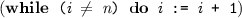, this method yields the following over-approximation of the reflexive transitive closure of F:

\begin{aligned} F^* \equiv n' = n \wedge i \le i' \wedge (i \le n \Rightarrow i' \le n) \end{aligned}

If we suppose that i is initially 0 and n is initially 100, then this formula implies the loop invariant that n is equal to 100, and i is in the interval [0, 100].### Example 5

(Recurrence analysis [4, 27]). Let $$F(X,X')$$ be a transition formula, and let $$\mathbf {x}$$ and $$\mathbf {x}'$$ denote vectors containing the variables X and $$X'$$, respectively. A linear recurrence inequation of F is a formula of the form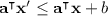that is entailed by F. The idea behind recurrence analysis is to extract a set of linear recurrence inequations for a formula,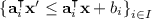, and to use the closed form of those recurrences to over-approximate the transitive closure of F: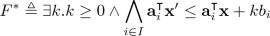For instance, consider the following loop:

The loop exhibits the following recurrences

which yields the following transition formula that summarizes the loop:

\begin{aligned} \exists k. k \ge 0 \wedge (2x' - y') \le (2x - y') - k \wedge y' \le y - k \wedge -y' \le -y + 3k\ . \end{aligned}

The loop also exhibits other recurrences (such as $$x' \le x - 1$$); however, the three selected recurrences are complete in the sense that all implied recurrences are non-negative linear combinations of these three (e.g., $$x' \le x - 1$$ is obtained by adding 1/2-times the first and second recurrences).

Such a complete set of recurrences exists for any transition formula F, which can be computed as follows. First, observe that the set of linear recurrences of F,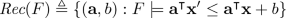is closed under non-negative linear combinations (i.e., it is a convex cone). Our goal is to find a (finite) set of generators for $$\textit{Rec}(F)$$—a finite set $$\left\{ (\mathbf {a}_i,b_i) \right\} _{i\in B}$$ such that

\begin{aligned} \textit{Rec}(F) = \left\{ (0, \lambda _0) + \sum _{i \in B} \lambda _i(\mathbf {a}_i, b_i) : \lambda _0 \ge 0, \lambda _i \ge 0 \text { for all } i \in B \right\} \ . \end{aligned}

To compute generators for $$\textit{Rec}(F)$$, we first introduce a fresh set of “difference” variables, $$\left\{ \delta _x \right\} _{x \in X}$$ and form a formula

\begin{aligned} \varDelta (F) \triangleq \exists X,X'. F \wedge \bigwedge _{x \in X} \delta _x = x' - x \ . \end{aligned}

Observe that $$(\mathbf {a},b) \in \textit{Rec}(F)$$ if and only if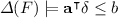. Thus, a set of generators for $$\textit{Rec}(F)$$ corresponds exactly to a half-space representation for the convex hull of $$\varDelta (F)$$, which can be computed using the algorithm from .

The class of linear recurrence inequations considered in this example can be generalized in various ways to yield more powerful invariant generation procedures. In particular,

•  computes linear recurrences with polynomial closed forms

•  computes polynomial recurrences with polynomial and complex exponential closed forms.

•  computes polynomial recurrences with polynomial and rational exponential closed forms.### 2.2 Weak Interpretations

Transition formulas are an appealing basis for algebraic program analysis, since all the operators (except the iteration operator) are precise—they simply encode the meaning of the program into logic. The significance of this is that transition formula algebras delay precision loss as long as possible, which helps to overcome loss of contextual information. However, there are algebraic analyses of interest that are defined on weak logical fragments that cannot precisely express union and/or relational composition.

### Example 6

(Affine relation analysis ). An affine relation is a relation that corresponds to the set of models of a transition formula of the form $$A\mathbf {x}' = B\mathbf {x} + c$$. Define the algebra of affine transition relations to be the regular algebra where the universe is the set of affine transition relations, 0 is interpreted as the empty relation, 1 is interpreted as the identity relation, $$+$$ is interpreted as the affine hull of $$R_1 \cup R_2$$ (the smallest affine relation that contains both $$R_1$$ and $$R_2$$), $$\cdot$$ is interpreted as relational composition, and $$*$$ is interpreted as the operation that sends any affine relation R to the limit of the sequence $$\{R_i\}_{i=0}^\infty$$ defined by

$$R_0 = 0 R_{i+1} = R_i + (R_i \cdot R) \text { for } i \ge 0$$

Since we have $$R_0 \subseteq R_1 \subseteq \dots$$ and if any $$R_{i+1}$$ properly contains $$R_i$$ the dimension of $$R_{i+1}$$ is strictly greater than that of $$R_i$$, this sequence must stabilize in finite time, so the operation $$R^*$$ is computable.## 3 Semantic Foundations

This section presents a general view of algebraic program analysis, with the goal of elucidating its underlying principles so that they may be understood outside the setting of graphs and regular expressions. This sets the stage for Sect. 4 and Sect. 5, wherein we will develop program analysis schemes that follow the same general “recipe” that we lay out in this section, but deviate from the instance of this recipe that we saw in Sect. 2.

Following the theory of abstract interpretation , we begin with a concrete semantics that defines the meaning of a program. The concrete semantics is specified as the least (or greatest) solution to a system of recursive equations. The concrete semantics is not computable—the goal of a program analysis is to approximate it. The way that this is accomplished in an algebraic analysis is by symbolically computing a closed-form solution to the semantic equations (i.e., a non-recursive system of equations whose (unique) solution coincides with the concrete semantics), and then interpreting that closed-form solution in an algebraic structure that approximates the algebra of the concrete semantics.

### 3.1 Semantic Equations

Given a control flow graph G, we can syntactically derive a system of equations E(G)—see Fig. 2. For each vertex v, we introduce a variable $$X_v$$ and an equation $$(X_v = R_v)$$ that relates that variable to the variables for v’s predecessors. Notice that this system of equations can be viewed as a (left-)regular grammar, with each non-terminal symbol $$X_v$$ recognizing the set of paths from the root r to the vertex v. This is an instance of the more general concept of a solution to a system of equations over an algebraic structure. A solution to the system of equations $$E(G) = \left\{ X_v = R_v \right\} _{v \in V}$$ over a regular interpretation $$\mathscr {I} = \left\langle \mathbf {A},f \right\rangle$$ is a function $$\sigma$$ that maps each variable to an element of $$\mathbf {A}$$ such that each equation is satisfied: for each equation $$(X_v = R_v)$$ in E(G), we have $$\sigma (X) = \mathscr {I}_\sigma \llbracket {R}\rrbracket$$, where $$\mathscr {I}_\sigma$$ is the interpretation obtained by extending the semantic function to variables by interpreting them according to $$\sigma$$.

The prototypical concrete semantics of interest in algebraic analysis is the relational semantics. The relational semantics of a program associates to every control flow vertex v a reachability relation $$R_v$$, which is the set of pairs $$\left\langle s,s' \right\rangle$$ such that if the program begins at r in state s, then it may reach v with state $$s'$$. The relational semantics may be obtained as the least solution to the system of semantic equations over the relational interpretation, which is defined as follows. The regular algebra of state relations, $$\mathbf {R}$$, has binary relations on states as its universe, 0 is interpreted as the empty relation $$\emptyset$$, 1 is interpreted as the identity relation $$\left\{ \left\langle s,s \right\rangle : s \in \textsf {State} \right\}$$, $$\cdot$$ is interpreted as relational composition, $$+$$ as union, and $$*$$ as reflexive, transitive closure. The relational interpretation $$\mathscr {R}$$ is the interpretation over the regular algebra of state relations where the semantic function maps each command to its associated transition relation; e.g.,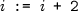is associated with the set of all pairs $$\left\langle s,s' \right\rangle$$ such thatand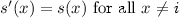. The relational semantics of a CFG G is the least solution to E(G) over the relational interpretation.

Having formulated the concrete semantics as the solution to a system of equations, we must now solve the system symbolically. The classical algorithm is a variation of Gaussian elimination, given in Algorithm 1. This algorithm is essentially Kleene’s algorithm  for computing a regular expression for a finite state automaton, recast in the language of equations. The front-solving step eliminates variables one-by-one, at each step i producing a system of equation of equations that is equivalent to the original, but in which the variable $$X_i$$ does not appear in the right-hand-side of any equations $$X_j = R_j$$ for $$j \ge i$$. The back-solving step eliminates all variable occurrences from right-hand-sides, at each step replacing $$X_i$$ with its closed form $$R_i$$ in each equation $$X_j=R_j$$ for $$j < i$$. An example illustrating the result of solving the system of equations in Fig. 2b symbolically appears in Fig. 2c. The significant difference to the familiar Gaussian elimination algorithm in linear algebra is the “loop-solving” step, which solves a single recursive equation $$X_i = R_i$$ symbolically by re-arranging $$R_i$$ into the form $$X_iA + B$$ and taking $$BA^*$$ to be the solution. The loop-solving step is justified under the relational interpretation, and more generally for any interpretation over a Kleene algebra.Footnote 3

### Definition 1

Let $$\mathbf {A} = \left\langle A, +, \cdot , *, 0, 1 \right\rangle$$ be a regular algebra. We say that $$\mathbf {A}$$ is an idempotent semiring if it satisfies the following (for all $$a,b,c, \in A$$):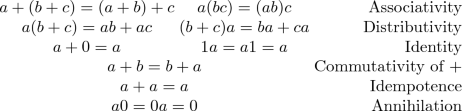In any idempotent semiring, we may define a natural order $$\le$$, where $$a \le b$$ iff $$a + b = b$$. Note that $$+$$ is the least upper bound with respect to this order.

We say that $$\mathbf {A}$$ is a Kleene algebra if it is an idempotent semiring and the following hold (for all $$a,x \in A$$):

$$\begin{array}{c@{}c@{}r} 1 + a(a^*) = a^* &{} 1+(a^*)a = a^* &{} \text {Unfolding}\\ ax\le x \Rightarrow a^*x \le x &{} xa \le x \Rightarrow xa^* \le x &{} \text {Induction} \end{array}$$

### Exercise 1

Show that in any Kleene algebra, the least solution to a (left-)linear recursive equation $$X = a + Xb$$ exists and is equal to $$ab^*$$

The sense in which Gaussian elimination computes a “closed-form solutions” to a system of left-linear equations E is that:

• (closed form) the right-hand sides do not refer to variables, and

• (solution) for any interpretation $$\mathscr {I}$$ over a Kleene algebra, for each equation $$(X=R) \in E$$, we have $$\sigma (X) = \mathscr {I}\llbracket {R}\rrbracket$$ where $$\sigma$$ is the least solution to E over $$\mathscr {I}$$.

The connection between Gaussian elimination and graph algorithms like Floyd-Warshall inspired Tarjan’s path-expression algorithm . In the language of graphs, Tarjan’s algorithm computes for each vertex v of a control flow graph G with root r a path expression $$\textit{PathExp}_{G}(r,v)$$ that recognizes the set of paths from r to v; in the language of equations, it solves left-linear systems of equations symbolically. Tarjan’s algorithm is preferred to Gaussian elimination in practice: is more efficient (nearly linear time for reducible flow graphs, compared to cubic time for Gaussian elimination) and produces simpler solutions. For expository purposes, we will continue to refer to Gaussian elimination for solving systems of equations, viewing Tarjan’s method as an efficient variation.

### 3.2 Abstract Interpretation

Gaussian elimination can solve a system of left-linear equations over a Kleene algebra (e.g., relational semantics) symbolically. However, the solution cannot be interpreted in the concrete algebra, since operators are not effective (that is, they cannot be implemented by a machine). We approximate the concrete semantics by interpreting the closed-form solution in an effective abstract algebra (e.g., one of the transition-formula algebras from Sect. 2).

Following the theory of abstract interpretation , the correctness of this approach is justified by establishing a relationship between the “concrete” and “abstract” interpretations. In the algebraic framework, a natural way to express the relationship is via a soundness relation , which is a binary relation between two algebras that is preserved by the operations of the algebra. Membership of a (concrete, abstract) pair in the relation indicates that the concrete element is approximated by the abstract element.

### Definition 2 (Soundness relation)

Given two $$\varSigma$$-interpretations $$\mathscr {I}^\natural = \left\langle \mathbf {A}^\natural ,f^\natural \right\rangle$$ and $$\mathscr {I}^\sharp = \left\langle \mathbf {A}^\sharp ,f^\sharp \right\rangle$$, $$- \Vdash - \subseteq A^\natural \times A^\sharp$$ is a soundness relation if $$f^\natural (a) \Vdash f^\sharp (a)$$ for all $$a \in \varSigma$$ and $$\Vdash$$ is a sub-algebra of the product algebra $$\mathbf {A}^\natural \times \mathbf {A}^\sharp$$; i.e., $$0^\natural \Vdash 0^\sharp$$, $$1^\natural \Vdash 1^\sharp$$, and for all $$x_1 \Vdash y_1$$ and $$x_2 \Vdash y_2$$ we have

• $$x_1 +^\natural x_2 \Vdash y_1 +^\sharp y_2$$

• $$x_1 \cdot ^\natural x_2 \Vdash y_1 \cdot ^\sharp y_2$$

• $$x_1^{*^\natural } \Vdash y_1^{*^\sharp }$$

The definition of soundness relation generalizes to interpretations over other classes of algebraic structures in the natural way: it is a binary relation over two algebras of the same signature that is preserved by every operation in the signature.

### Example 7

(Transition formula overapproximation). Let $$\mathbf {R}$$ denote the algebra of state relations and $$\mathbf {TF}$$ denote an algebra of transition formulas. The over-approximation relation is defined by

$$R \Vdash _O F \iff \forall \left\langle s,s' \right\rangle \in R, s \rightarrow _F s'.$$

Preservation of constants and the sequencing and choice operations is easily verified; to show that $$\Vdash _O$$ is a soundness relation, we need only to show that $$R \Vdash _O F$$ implies $$R^{*^\mathbf {R}} \Vdash _O F^{*^{\mathbf {TF}}}$$; i.e., $$(-)^{*^{\mathbf {TF}}}$$ over-approximates reflexive transitive closure. Of course, this proof depends on the particular implementation of the iteration operator.

The over-approximate soundness relation allows us to verify safety properties: if $$R \Vdash _O F$$ and F entails some property P, then R satisfies P.### Example 8

(Transition formula underapproximation). The under-approximation relation is defined by

$$R \Vdash _U F \iff \forall s,s' . s \rightarrow _F s' \Rightarrow \left\langle s,s' \right\rangle \in R,$$

Preservation of constants and the sequencing and choice operations is again easily verified; to show that $$\Vdash _U$$ is a soundness relation, we need only to show that $$R \Vdash _O F$$ implies $$R^{*^\mathbf {R}} \Vdash _O F^{*^{\mathbf {TF}}}$$; i.e., $$(-)^{*^{\mathbf {TF}}}$$ under-approximates reflexive transitive closure. The iteration operators in Sect. 2 are all over-approximate. An example of an under-approximate iteration operator is

\begin{aligned} F^* \triangleq \bigvee _{i = 0}^n \underbrace{F \circ \dots \circ F}_{i \textit{ times}} \end{aligned}

(for some fixed choice of n) which corresponds to bounded model checking , with an unrolling bound of n.

The under-approximate soundness relation allows us to refute safety properties: if $$R \Vdash _U F$$ and F does not entail some property P, then R does not satisfy P.The problem of “approximating the behavior of a program” can be formalized as follows:

Given a system of semantic equations over a set of variables $$\mathcal {X}$$ describing the concrete semantics of a program (i.e., its least solution $$\sigma ^\natural$$ over some interpretation $$\mathscr {I}^\natural$$), find some $$\sigma ^\sharp : \mathcal {X} \rightarrow \mathbf {A}^\sharp$$ such that for each variable $$X \in \mathcal {X}$$, we have $$\sigma ^\natural (X) \Vdash \sigma ^\sharp (X)$$.

The algebraic approach to this problem is to compute for each variable X a closed form $$R_X$$ (such that $$\sigma ^\natural (X) = \mathscr {I}^\natural (R_X)$$), and define $$\sigma ^\sharp (X) \triangleq \mathscr {I}^\sharp (R_X)$$. The correctness of this approach is justified by the following soundness lemma, which follows by induction on regular expressions.

### Lemma 1 (Soundness)

Let $$\varSigma$$ be an alphabet, let $$\mathscr {I}^\natural = \left\langle \mathbf {A}^\natural ,f^\natural \right\rangle$$ and $$\mathscr {I}^\sharp = \left\langle \mathbf {A}^\sharp ,f^\sharp \right\rangle$$ be $$\varSigma$$-interpretations, and let $$\Vdash \subseteq A^\natural \times A^\sharp$$ be a soundness relation. Then for any regular expression $$R \in \textsf {RegExp}(\varSigma )$$, we have $$\mathscr {I}^\natural \llbracket {R}\rrbracket \Vdash \mathscr {I}^\sharp \llbracket {R}\rrbracket$$

### 3.3 Discussion

A subtlety of algebraic program analysis is that most algebras of interest in program analysis are not Kleene algebras (for instance, none of the algebras in Sect. 2 are), and so in general, Gaussian elimination does not find solutions to systems of equations over “abstract” interpretations corresponding to program analyses. This technical difficulty is sidestepped by appealing to the concrete semantics (which typically is defined over a Kleene algebra, such as the algebra of state relations) to justify the use of path-expression algorithms, and a sound approximating algebra to interpret the resulting expressions. The fact that the abstract interpretation of the closed-form solution to the concrete system of equations does not yield a solution to the abstract system of equations is immaterial: our goal is to over-approximate the concrete rather than solve the abstract.

Formalizing a program analysis as an algebraic structure allows one to understand the behavior of program analyses in terms of algebraic laws, and use the language of algebra to reason about program analyses. For example, any transition formula algebra (in the family described in Sect. 2.1) is an idempotent semiring, and so any two $$*$$-free regular expressions that denote the same language have the same (up to logical equivalence) interpretation as a transition formula. While none of the iteration operators in Sect. 2.1 satisfy the Unfolding and Induction laws of Kleene algebra, they do satisfy weaker pre-Kleene algebra iteration laws:

$$\begin{array}{c@{}c@{}r} 1 \le a^* &{} \text {Reflexivity}\\ a \le a^* &{} \text {Extensivity}\\ a^*a^* = a^* &{} \text {Transitivity}\\ a \le b \Rightarrow a^* \le b^* &{} \text {Monotonicity}\\ \text {For any { n}, } (a^n)^* \le a^* &{} \text {Unrolling} \end{array}$$

A concrete use-case for these laws appears in , which develops regular expression transformation techniques that preserve concrete semantics but are guaranteed to produce (non-strictly) more precise abstract semantics.

Such laws can also be useful for users of program analysis tools. For example, since all operations are monotone (as a consequence of the monotonicity and idempotent-semiring laws), a user can rely on the principle that “more information in yields more information out.” If a user alters a program P by adding additional assume commands to get a program $$P'$$ (e.g., expressing invariants that are found by some other automated invariant generation technique, user-provided hints, etc.), monotonicity means that they may rely on the fact that the analysis will produce summaries for $$P'$$ that are at least as precise as those for P.

A Recipe for Algebraic Program Analysis. We conclude this section by presenting a general view of algebraic program analysis, abstracted from the language of graphs and regular expressions:

• 1. (Modeling) Express the concrete semantics as the least (or greatest) solution to a system of recursive equations (e.g., relational semantics as the least solution to the left-linear system of equations corresponding to a control flow graph).

• 2. (Closed forms) Design a suitable language of “closed-form solutions” and an algorithm for computing them (e.g., regular expressions and path-expression algorithms).

• 3. (Interpretation) Design an abstract interpretation of the language of closed forms and a soundness relation connecting the concrete and abstract interpretations (e.g., transition-formula algebras (Sect. 2.1) and the over-approximate soundness relation (Ex. 7)).

Section 4 and Sect. 5 give two more instances of this generic recipe, generalizing beyond left-linear equations and regular-expressions as closed forms. Section 4 considers linear equations (and an appropriate language of closed forms); Sect. 5 considers another form of equation with $$\omega$$-regular expressions as closed forms.

## 4 Interprocedural Analysis

Algebraic program analyses are oriented around computing summaries for program fragments, and are naturally suited to analyzing programs with procedures. Following Cousot & Cousot  and Sharir & Pnueli , the idea is to structure the analysis in two phases:

• Phase I: compute for each procedure X a summary that approximates the behavior of X (including the actions of all procedures called transitively from X).

• Phase II: analyze whole-program paths from the start of the main procedure, using the summaries to interpret procedure calls.

An example of a program with procedures is given in Fig. 3(a). The CFGs for its procedures are shown in Fig. 3(b) along with a set of equations corresponding to the CFGs (Fig. 3(c)). For Phase I, it is also useful to consider the following equations in which we have eliminated all variables except for those of the form $$X_{s,x}$$, which represent the procedure summaries.

\begin{aligned} \begin{array}{@{}l@{}c@{}l@{}} X_{s_1,x_1} &{}=&{} (\left\langle s_1,a \right\rangle \cdot X_{s_2,x_2} +\left\langle s_1,b \right\rangle ) \cdot X_{s_2,x_2} \\ X_{s_2,x_2} &{} = &{} X_{s_3,x_3} \cdot X_{s_3,x_3} \\ X_{s_3,x_3} &{} = &{} \left\langle s_3,x_3 \right\rangle \end{array} \end{aligned}
(1)

This system of equations can be obtained either by a process of successively eliminating variables from Fig. 3(c), or they can be read off directly from each control-flow graph: sequential composition corresponds to $$\cdot$$, and branching corresponds to $$+$$.

We can also construct a graph of the dependencies among the variables in the equation system. In this case, we would have

\begin{aligned} X_{s_3,x_3} \longrightarrow X_{s_2,x_2} \longrightarrow X_{s_1,x_1} \end{aligned}
(2)

(which is also isomorphic to the program’s call graph). Note that the equations in Eq. (1) are not left-linear. However, by eliminating variables in a topological order of Eq. (2), these systems can still be solved using Gaussian elimination (Algorithm 1).

\begin{aligned} \begin{array}{@{}l@{}c@{}l@{}} X_{s_3,x_3} &{} = &{} \left\langle s_3,x_3 \right\rangle \\ X_{s_2,x_2} &{} = &{} \left\langle s_3,x_3 \right\rangle \cdot \left\langle s_3,x_3 \right\rangle \\ X_{s_1,x_1} &{}=&{} (\left\langle s_1,a \right\rangle \cdot \left\langle s_3,x_3 \right\rangle \cdot \left\langle s_3,x_3 \right\rangle +\left\langle s_1,b \right\rangle ) \cdot \left\langle s_3,x_3 \right\rangle \cdot \left\langle s_3,x_3 \right\rangle \end{array} \end{aligned}
(3)

Unfortunately, this strategy breaks down for programs with recursive procedures: the essential difficulty is in computing the summaries of procedures that are directly recursive or part of a set of mutually recursive procedures. We will return to this issue shortly, after a brief discussion of Phase II, which can be addressed via algebraic program analysis, regardless of whether the original equation system contains recursion.

With closed-form solutions for the procedure summaries in hand, Phase II can be addressed with Gaussian elimination. (Note that for a program with recursive procedures, the transformed Phase II system is still recursive. However, it is left-recursive, and so can be handled with regular expressions, and analyzed using the transition-formula interpretations of Sect. 2—the “loops” in Phase II correspond to sequences of recursive calls). Figure 4 shows the equation system used for Phase II for the program from Fig. 3 in graphical form. The graph is similar to Fig. 3(b) with (i) additional edges from each call-site to the start node of the called procedure, and (ii) the edges previously labeled with “$$X_2$$” and “$$X_3$$” are now labeled with the values from Eq. (3) for the corresponding procedure summaries: $$\left\langle s_3,x_3 \right\rangle \cdot \left\langle s_3,x_3 \right\rangle$$ and $$\left\langle s_3,x_3 \right\rangle$$, respectively.

The remainder of this section focuses on Phase I: computing procedure summaries. Consider the two-procedure program shown in Fig. 5(a). CFGs for its procedures are shown in Fig. 5(b) along with a set of recursive equations corresponding to the interprocedural CFG. Unfortunately, equations like those in Fig. 5(c) do not fit naturally with the recipe given in Sect. 3.3. The essential difficulty is with item 3.3: “Design a suitable language of ‘closed-form solutions’ and an algorithm for computing them.” In particular, we cannot use regular expressions and path-expression algorithms because the equations in Fig. 5(c) are not left-linear (and they cannot be put in left-linear form).

Two ideas are involved in using algebraic program analysis to summarize recursive procedures:

1. 1.

The generalization by Esparza et al.  of Newton’s method—the classical numerical-analysis algorithm for finding roots of real-valued functions—to a method for solving a system of equations over a semiring $$\mathcal {S}$$, called Newtonian Program Analysis (NPA). As in its real-valued counterpart, each iteration of NPA solves a simpler “linearized” problem. (See Sect. 4.1.)

2. 2.

The technique of Reps et al.  for applying the algebraic-program-analysis recipe to the linearized problems that arise in NPA. (See Sect. 4.2.)

### 4.1 Motivation: Newtonian Program Analysis

To motivate why we are interested in the special case of linear equations (Sect. 4.2), this section provides a brief overview of how linear equations arise in NPA. Let $$E = \left\{ X_i = R_i \right\} _{i=1}^n$$ be a system of equations, and fix an interpretation $$\mathscr {I}$$ over some algebra $$\mathbf {A}$$. Define a function $$\mathbf {f} : A^n \rightarrow A^n$$ by $$\mathbf {f}(\sigma ) = (\mathscr {I}_\sigma \llbracket {R_1}\rrbracket ,\dots ,\mathscr {I}_\sigma \llbracket {R_n}\rrbracket )$$ (i.e., the n-tuple of interpreted right-hand-sides, where variables are interpreted according to $$\sigma$$). NPA is an iterative method for program analysis that solves the following sequence of problems for $$\mathbf {\nu }$$:(4)

where $$\mathbf {Y}^{(i)}$$ is the value of $$\mathbf {Y}$$ in the least solution of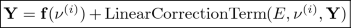(5)

Thus, NPA is similar to Kleene iteration, except that on each iteration, $$\mathbf {f}(\mathbf {\nu }^{(i)})$$ is “corrected” by an amount controlled by $$\text {LinearCorrectionTerm}(E,\mathbf {\nu }^{(i)},\mathbf {Y})$$—a function of $$\mathbf {f}$$, the current approximation $$\mathbf {\nu }^{(i)}$$, and (vector) variable $$\mathbf {Y}$$—which nudges the next approximation $$\mathbf {\nu }^{(i+1)}$$ in the right direction at each step.

The linear correction term is the result of replacing each right-hand side $$R_i = \sum _j R_j$$ with a sum $$\sum _{i=0}^n R_{i,j,k}$$, where each $$R_{i,j,k}$$ is obtained from $$R_{i,j}$$ by replacing all variables, except possibly one, with its interpretation in $$\nu$$. (The formal definition can be found elsewhere [26, §3.2].) For example, consider the system of equations below, a simplified variant of Fig. 5(c) that is obtained by eliminating all variables except $$X_{s_1,x_1},X_{s_2,b},X_{s_2,x_2}$$: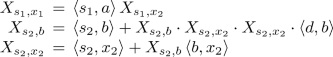(6)

The transformation results in the following system (for brevity, we denote $$Y_{s_1,x_1},Y_{s_2,b},Y_{s_2,x_2}$$ by $$Y_1,Y_2,Y_3$$):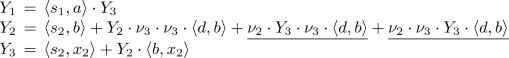(7)

Note that the two underlined summands are both truly linear: they are linear, but not left-linear nor right-linear.

The process of solving Eqs. (4) and (5) for $$\mathbf {\nu }^{(i+1)}$$, given $$\mathbf {\nu }^{(i)}$$, is called one Newton round. On the initial Newton round, we set $$\langle {\nu _1^{(0)}, \nu _2^{(0)}, \nu _3^{(0)}}\rangle \leftarrow \langle {{0}, \mathscr {I}\llbracket {\left\langle s_2,x_2 \right\rangle }\rrbracket , \mathscr {I}\llbracket {\left\langle s_3,x_3 \right\rangle }\rrbracket }\rangle$$. On round $$i+1$$, we solve Eq. (7) for $$\langle {Y_1, Y_2, Y_3}\rangle$$ with $$\langle {\nu _1, \nu _2, \nu _3}\rangle$$ set to the value $$\langle {\nu _1^{(i)}, \nu _2^{(i)}, \nu _3^{(i)}}\rangle$$ obtained on round i, and then set $$\langle {\nu _1^{(i+1)}, \nu _2^{(i+1)}, \nu _3^{(i+1)}}\rangle \leftarrow \langle {Y_1, Y_2, Y_3}\rangle$$.

Operationally, the linearization transformation imposes a particular protocol for sampling the program’s space of behaviors. For instance, in Fig. 5(b), the procedure $$X_2$$ has two call-sites along the loop through b. In Eq. (7), each right-hand-side summand in the equation for $$Y_2$$ has at most one variable: the transformation inserted $$\nu _2$$ or $$\nu _3$$ at various call-sites (considering $$X_{s_2,b}$$ as a pseudo-call-site corresponding to tail recursion), and left at most one variable $$Y_i$$ in each summand. In essence, during a given Newton round, the analyzer samples the behavior of $$\mathbf {f}$$ by taking the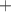of various paths through the transformation of $$\mathbf {f}$$. Along each path through a (transformed) right-hand side, the summary for each pseudo-call-site $$X_i$$ encountered is held fixed at $$\nu _i$$, except for possibly one pseudo-call-site on the path, which is explored by visiting (the linearized version of) the called procedure. The summaries $$\nu _1$$, $$\nu _2$$, $$\nu _3$$ are updated according to the result of this exploration, and the algorithm performs the next Newton round.

The analogy between NPA and Newton’s method in numerical analysis is that in both cases one creates a linear approximation of $$\mathbf {f}(\mathbf {X})$$ around the “point” $$(\mathbf {\nu }^{(i)}, \mathbf {f}(\mathbf {\nu }^{(i)}))$$; the solution of the linear system is the next approximation of $$\mathbf {X}$$.

### 4.2 Algebraic Program Analysis for Linear Equations

In this section, we instantiate the recipe for algebraic program analysis from Sect. 3.3 to solve a system of linear equations, such as the linearized problems that arise as Eq. (5) . This goal may seem out of reach because item 3.3 of the recipe requires us to “design a suitable language of ‘closed-form solutions’ and an algorithm for computing them.”

What is a suitable language of closed-form solutions of linear equations? Clearly the regular expressions and path-expression algorithms used in Sect. 2 and Sect. 3 will not do, because the least solution under the language interpretation to the (truly) linear equation $$X = aXb + 1$$ is $$\left\{ a^i b^i : i \ge 0 \right\}$$, which is the canonical example of a linear-context-free language that is not regular. However, over fifty years ago, formal-language theorists established that linear-context-free languages have certain similarities to regular languages [17, 34, 61], and we can make use of this property to design a language of closed forms for linear equations. Intuitively, $$\left\{ a^i b^i : i \ge 0 \right\}$$ can be obtained by (i) introducing paired alphabet symbols, such as (ab), (ii) defining concatenation of paired symbols as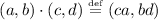, (iii) defining Kleene-star in the natural way over paired-symbol concatenation, so $$(a,b)^*$$ is the language of paired words $$\left\{ (a^i, b^i) : i \ge 0 \right\}$$, and (iv) applying an operation that concatenates the left word and right word of each paired word: $$\left\{ (a^i, b^i) : i \ge 0 \right\} \mapsto \left\{ a^i b^i : i \ge 0 \right\}$$.

For the purpose of algebraic program analysis, this idea can be formalized by introducing tensored regular expressions over an alphabet $$\varSigma$$, whose syntax is defined as follows:Footnote 4

\begin{aligned} a \in \varSigma \\ R \in \textsf {RegExp}(\varSigma )&\,::\,= a \mid 0 \mid 1 \mid R_1 + R_2 \mid R_1 \cdot R_2 \mid R^* \mid S^\lightning \\ S \in \textsf {RegExp}_T(\varSigma )&\,::\,= R_1 \otimes R_2 \mid \underline{0} \mid \underline{1} \mid S_1 \oplus S_2 \mid S_1 \odot S_2 \mid S^\circledast \end{aligned}

We can now follow the pattern of Sect. 2, and define algebras suitable for interpreting tensored regular expressions.

### Definition 3

A tensor-product algebra $$\mathcal {T}= \left\langle \mathbf {A},\mathbf {T},\otimes ,\lightning \right\rangle$$ consists of two regular algebras $$\mathbf {A}$$ and $$\mathbf {T}$$ along with an operation $$\otimes : A \times A \rightarrow T$$, called tensor product, and an operation $$\lightning : T \rightarrow A$$, called detensor.

### Example 9

(Standard interpretation). The standard interpretation from Example 1 can be extended to tensored regular expressions by defining a universe of languages over word pairs (“tensored words”) $$T = 2^{\varSigma ^* \times \varSigma ^*}$$, whose operators are given by: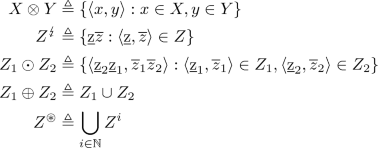Note that this interpretation allows tensored regular expressions to be used to capture linear context-free languages. For instance, the equation $$X = aXb + 1$$, whose least solution is $$\left\{ a^i b^i : i \ge 0 \right\}$$ can be written in closed form as $$X = ((a \otimes b)^\circledast )^\lightning$$, and the equation $$X = aXa + bXb + 1$$, whose least solution is the language of even-length palindromes over $$\{a,b\}$$, can be written as $$X = (((a \otimes a) \oplus (b \otimes b))^\circledast )^\lightning$$.### Example 10

(Relational interpretation). The relational interpretation can be extended to tensored regular expressions by defining an algebra of binary state-pair relations, as follows.Footnote 5 The universe is the set of relations on $$\textsf {State}\times \textsf {State}$$ (i.e., an element of the universe is a subset of $$\textsf {State}\times \textsf {State}\times \textsf {State}\times \textsf {State}$$). Comparing with the standard interpretation, (in which an element $$\left\langle p_1,p_2 \right\rangle$$ consists of a “backwards path” p and a “forwards continuation”) we may think of an element $$\left\langle \begin{pmatrix}s_1'\\ s_2\end{pmatrix}, \begin{pmatrix}s_1\\ s_2'\end{pmatrix} \right\rangle$$ of a state-pair relation as consisting of two pre/post state pairs: a “backwards” pair $$s_1' \;^*\leftarrow s_1$$ and a “forwards” pair $$s_2 \rightarrow ^* s_2'$$. In the algebra of state-pair relations, 0 is interpreted as the empty relation, 1 as the identity relation, and $$+$$ as union. The remaining operators are given by:

\begin{aligned} R_1 \otimes R_2&= \left\{ \left\langle \begin{pmatrix}s_1'\\ s_2\end{pmatrix},\begin{pmatrix}s_1\\ s_2'\end{pmatrix} \right\rangle : \left\langle s_1,s_1' \right\rangle \in R_1, \left\langle s_2,s_2' \right\rangle \in R_2 \right\} \nonumber \\ T^\lightning&= \left\{ \left\langle s,s' \right\rangle : \exists s'',s''. \left\langle \begin{pmatrix}s''\\ s'''\end{pmatrix},\begin{pmatrix}s\\ s'\end{pmatrix} \right\rangle \in T \wedge s'' = s''' \right\} \\ T_1 \odot T_2&= \left\{ \left\langle \begin{pmatrix}s_1\\ s_2\end{pmatrix},\begin{pmatrix}s_1'\\ s_2'\end{pmatrix} \right\rangle : \left\langle \begin{pmatrix}s_1\\ s_2\end{pmatrix},\begin{pmatrix}s_1''\\ s_2''\end{pmatrix} \right\rangle \in T_1 \wedge \left\langle \begin{pmatrix}s_1''\\ s_2''\end{pmatrix},\begin{pmatrix}s_1'\\ s_2'\end{pmatrix} \right\rangle \in T_2 \nonumber \right\} \\ T^\circledast&= \bigcup _{i=0}^\infty \underbrace{T \odot \dots \odot T}_{i \text { times}} \nonumber \end{aligned}
(8)

Note that the tensored sequencing operation is just a form of relational composition (over tuples of stacked elements); similarly, tensored iteration is a form of reflexive transitive closure.### Example 11

(Transition-formula interpretation). Transition formulas can be used to interpret tensored regular expression in a way analogous to the relational interpretation (as one should expect, because there must be a soundness relation between them!). A tensored transition formula T is a formula over four vocabularies, representing the value of the variables before and after a pair of computations. The tensor and detensor operations are essentially the same as those from the relational interpretation, translated into logic:

\begin{aligned} (F_1 \otimes F_2)\left( \begin{pmatrix}X_1'\\ X_2\end{pmatrix}, \begin{pmatrix}X_1\\ X_2'\end{pmatrix} \right)&\triangleq F_1(X_1,X_1') \wedge F_2(X_2,X_2') \\ T^\lightning (X,X')&\triangleq \exists \begin{pmatrix}Y_1\\ Y_2\end{pmatrix}. T\left( \begin{pmatrix}Y_1\\ Y_2\end{pmatrix}, \begin{pmatrix}X\\ X'\end{pmatrix} \right) \wedge Y_1 = Y_2 \nonumber \end{aligned}
(9)

In the Eq. (9), the vocabularies $$X_1$$, $$X_1'$$, $$X_2$$, and $$X_2'$$ track the original role of the respective vocabulary in $$F_1$$ or $$F_2$$. The “stacked” notation is intended to be suggestive of an interpretation of a tensored transition formula over a doubled vocabulary, where the variables are $$X_1' \cup X_2$$ and their “primed copies” are $$X_1 \cup X_2'$$. To make the connection with Sect. 2.1 more apparent, we shall define $$W_1=X_1'$$, $$W_2=X_2$$, $$W_1'=X_1$$, $$W_2'=X_2'$$. With this notation, the product operation can be defined as:

$$(T_1 \odot T_2)\left( \begin{pmatrix}W_1\\ W_2\end{pmatrix}, \begin{pmatrix}W_1\\ W_2\end{pmatrix}' \right) \triangleq \exists \begin{pmatrix}W_1\\ W_2\end{pmatrix}'' . T_1\left( \begin{pmatrix}W_1\\ W_2\end{pmatrix}, \begin{pmatrix}W_1\\ W_2\end{pmatrix}'' \right) \wedge T_2\left( \begin{pmatrix}W_1\\ W_2\end{pmatrix}'', \begin{pmatrix}W_1\\ W_2\end{pmatrix}' \right)$$

As with the relational interpretation, the product operation is just a form of relational composition (over tuples of stacked elements).

Remarkably, the algebra of tensored transition formulas is the same as the algebra of untensored transition formulas, just over an extended set of variables. In particular, the iteration operators from Sect. 3 can be used to implement $$\circledast$$. For instance, consider the recursive procedure

The path to the recursive call of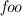and the path from the recursive call to exit can be modeled by the transition formulas F and G, respectively:

\begin{aligned} F&\triangleq a' = a + 1 \wedge b' = b\\ G&\triangleq b' = b + 1 \wedge a' = a \end{aligned}

A procedure summary for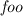can be calculated by evaluating $$((F \otimes G)^\circledast )^\lightning$$, using recurrence analysis (Example 5) to implement the $$\circledast$$ operator:

We now show how to compute closed forms for linear equations. First, we perform a regularizing transformation, which takes a system of linear equations $$E_\text {Lin}$$ and converts it into a system of left-linear equations $$E_\text {LeftLin}$$. The transformation takes each right-hand-side term of the form $$a \cdot Y \cdot b$$ and converts it to $$Z \odot (a \otimes b)$$, where Y and Z are variables whose values are elements of the regular algebras $$\mathbf {A}$$ and $$\mathbf {T}$$ of a tensor-product algebra $$\left\langle \mathbf {A},\mathbf {T},\otimes ,\lightning \right\rangle$$.

### Definition 4

Given a linear equation system $$E_\text {Lin}$$ over the regular algebra $$\mathbf {A}$$ of a tensor-product algebra $$\mathcal {T}= \left\langle \mathbf {A},\mathbf {T},\otimes ,\lightning \right\rangle$$, the regularizing transformation $$\tau _\text {Reg}$$ creates a left-linear equation system $$E_\text {LeftLin}= \tau _\text {Reg}(E_\text {Lin})$$ over $$\mathbf {T}$$ by transforming each equation of $$E_\text {Lin}$$ as follows:

where $$Z_i$$ and $$Z_j$$ are variables that take on values from $$\mathbf {T}$$.

For instance, if the regularizing transformation is applied to the linear system of equations in Fig. 6a, the result is the system of equations Fig. 6b. Because Fig. 6b is left-linear, we can now use the approach from Sect. 2 and Sect. 3—that is, create a closed-form solution for each variable $$Z_i$$ by finding a path expression for the variable in the graph Fig. 6c. Finally, one gives a closed-form solution for each variable $$Y_i$$ for the linear equation system in Fig. 6a by applying $$(-)^\lightning$$ to each path expression—see Fig. 6d. This algorithm for computing closed-form solutions to linear equations is justified in the tensored-relational interpretation, and more generally, in any interpretation whose algebra forms what we dub a Kronecker algebra, defined as follows:

### Definition 5

A Kronecker algebra $$\mathbf {Kr} =$$ $$\langle \left\langle A, +, \cdot , *, 0, 1 \right\rangle$$, $$\left\langle T, \oplus , \odot , \circledast , \underline{0}, \underline{1} \right\rangle$$, $$\otimes , \lightning \rangle$$ is a tensor-product algebra that consists of two Kleene algebras $$\left\langle A, +, \cdot , *, 0, 1 \right\rangle$$ and $$\left\langle T, \oplus , \odot , \circledast , \underline{0}, \underline{1} \right\rangle$$ such that (i) the natural order forms a complete lattice (i.e., both algebras have all infinite sums), and (ii) the following properties hold:

1. 1.

$$0 \otimes 0 = \underline{0}$$

2. 2.

$$1 \otimes 1 = \underline{1}$$

3. 3.

$$(a \otimes b)^\lightning = a \cdot b$$, for all $$a, b \in A$$

4. 4.

$$(a_1 \otimes b_1) \odot (a_2 \otimes b_2) = (a_2 \cdot a_1) \otimes (b_1 \cdot b_2)$$, for all $$a_1, a_2, b_1, b_2 \in A$$

5. 5.

$$(t_1 \oplus t_2)^\lightning = t_1^\lightning +t_2^\lightning$$, for all $$t_1, t_2 \in T$$

We assume that all distributivity properties of A and T, as well as item 5, hold for infinite sums. In particular, for item 5, we have

\begin{aligned} \left( \bigoplus _{i \in I} t_i \right) ^\lightning = \sum _{i \in I} t_i^\lightning \end{aligned}
(10)

### 4.3 Discussion

The Instantiation of the Recipe. Returning to the recipe from Sect. 3.3, what we have done for a system of linear equations $$E_\text {Lin}$$ is to instantiate the recipe as follows:

1. 1.

(Modeling). The concrete semantics is the least solution of $$E_\text {Lin}$$ interpreted in relational semantics.

2. 2.

(Closed forms). Each variable of $$E_\text {Lin}$$ is expressed as the detensor ($$(-)^\lightning$$) of a tensored regular expression. Closed forms are computed from the closed-forms of the left-linear system of equations $$\tau _\text {Reg}(E_\text {Lin})$$ that results from the regularizing transformation (e.g., see Fig. 6).

3. 3.

(Interpretation). Tensored regular expressions can be interpreted as tensored transition formulas (Example 11), which are simply transition formulas over a “doubled” vocabulary.

Two Lessons. We would like to mention two lessons that we learned while working on this material over the years.

1. 1.

For the problems that arise in NPA, we must solve an equation system that is truly linear, not left-linear or right-linear. A reasonable sanity check might go as follows:

• Algebraic program analysis à la Sect. 2 solves a left-linear (or right-linear) system of equations using methods based on regular expressions.

• NPA repeatedly creates a system of linear equations that needs to be solved. Such linear equations are related to linear context-free languages, such as the language $$\{ a^i b^i \}$$, which is not regular.

• Ergo, it is a non-starter to attempt to apply algebraic program analysis to the equations that arise on each round of NPA.

However, as shown in this section, it was possible to side-step this fundamental mismatch, by extending algebraic program analysis to systems of linear equations using Kronecker algebras, which have additional operations, such as tensor product and detensor.

Thus, beyond the technical details, perhaps a more important takeaway is “be careful how you apply sanity checks.” There is a risk that a plausible-sounding sanity check could cause you to discard an idea that is worth pursuing.

2. 2.

In some sense, the solution using Kronecker algebras goes against the grain of what computer scientists typically preach, namely, create appropriate abstractions (in the sense of abstract data-types) for a problem at hand, and then program your solution, thinking of the chosen abstractions as the operations of an abstract machine. This style of thinking is considered central to managing complexity in computer science, and it is generally considered heresy to break an abstraction.

For algebraic program analysis, the abstraction is regular algebra, used with interpretations that are abstractions (in the sense of abstract interpretation ) of a program’s concrete transition relations. However, the introduction of tensor product and detensor breaks that abstraction! To understand what we mean, consider the definition of $$F \cdot G$$ for transition relations in Boolean programs, i.e.,

$$(F \cdot G)(W,Z) \triangleq \exists X,Y . F(W,X) \wedge G(Y,Z) \wedge (X = Y),$$

and the definitions of $$F \otimes G$$ and $$T^\lightning$$,Footnote 6 namely,

$$\begin{array}{rcl} (F \otimes G)(W,X,Y,Z) &{} \triangleq &{} F(W,X) \wedge G(Y,Z) \\ T(W,X,Y,Z)^\lightning &{} \triangleq &{} \exists X,Y . T(W,X,Y,Z) \wedge (X = Y) \end{array}$$

The product operation $$F \cdot G$$ has three distinct steps: (i) conjoin F(WX) and G(YZ); (ii) conjoin the equality $$X = Y$$; and (iii) project out vocabularies X and Y. In essence, tensor product and detensor break the abstraction of $$\cdot$$ as an indivisible operation: $$\cdot$$ is decomposed into two more-granular operations, $$\otimes$$ and $$\lightning$$. By performing $$F \otimes G$$, we perform just the first step of $$\cdot$$, and only later, when $$\lightning$$ is performed, do we “finish up” by applying the second and third steps of $$\cdot$$. The advantage is that we can operate on tensored values for some number of steps before “finishing” some earlier $$\cdot$$.

Again, beyond the technical details, the takeaway may be the process that we went through, which may be of value as a conceptual tool in other contexts:

• The insight on how to break the abstraction—both as presented here and as occurred during our research seven or eight years ago—came from thinking about one specific interpretation of Kleene algebra: transition relations for Boolean programs.

• The algebraic properties of the new, finer-granularity operations allowed us to abstract out a new algebra, dubbed in this paper Kronecker algebra.

• The ideas could now be applied in other contexts by finding other interpretations of Kronecker algebra (or, because we are interested in program analysis, by finding interpretations that over-approximate Kronecker algebra).

## 5 Termination Analysis

This section describes how algebraic program analysis can be applied to termination analysis, based on the approach of . The goal of termination analysis is to prove that a program has no infinite executions. Our high-level strategy is to exploit compositionality: we prove that a loop terminates by first computing a summary (e.g., a transition formula) for its body, and then finding a termination argument for the summary.

Following Sect. 3, we first formalize a concrete semantics as the (greatest) solution of a system of semantic equations. An appropriate notion of concrete semantics for termination analysis is the set of non-terminating states of the program (from which there exists an infinite execution)—the program terminates exactly when none of the program’s initial states belong to this set. As in Sect. 3, this system of equations can be derived syntactically from a program’s control flow graph—see Fig. 7 for an example. The non-terminating states of the program are the greatest solution to this system of equations over the algebra where the universe is the set of states, $$\boxplus$$ is interpreted as union (a state is non-terminating if it has at least one infinite execution) and $$\boxdot$$ is interpreted as preimage (a state is non-terminating iff it can reach a non-terminating state).Footnote 7

A suitable language of “closed-form solutions” for the system of equations that arise in termination analysis is $$\omega$$-regular expressions. The syntax of $$\omega$$-regular expressions over an alphabet $$\varSigma$$ is as follows: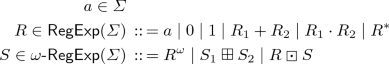The semantics of a ($$\omega$$)-regular expressions is given by an interpretation over an $$\omega$$-algebra and a regular algebra.

### Definition 6

An $$\omega$$-algebra over a regular algebra $$\mathbf {A}$$ is 4-tuple $$\mathbf {B} = \left\langle B,\boxdot ^B,\boxplus ^B,^{\omega ^B} \right\rangle$$ consisting of a universe B, an operation $$\boxdot ^B : A \times B \rightarrow B$$, an operation $$\boxplus ^B : B \times B \rightarrow B$$, and an operation $$(-)^{\omega ^B} : A \rightarrow B$$.

### Example 12

(Standard interpretation). In the standard interpretation of $$\omega$$-regular expressions, the universe consists of sets of infinite sequences over the alphabet $$\varSigma$$, and the operations are

\begin{aligned} W_1 \boxplus W_2&\triangleq W_1 \cup W_2&\text {Union}\\ X \boxdot W&\triangleq \left\{ xw : x \in X, w \in W \right\}&\text {Concatenation}\\ X^\omega&\triangleq \left\{ x_1x_2 \dots : x_1,x_2,\dots \in X \right\}&\text {Infinite repetition} \end{aligned}

For example, an $$\omega$$-regular expression that recognizes all infinite paths in Fig. 7a starting at r is:### Example 13

(Nonterminating state interpretation). The non-terminating state algebra is an $$\omega$$-algebra over the algebra of state relations. Its universe consists of sets of states. The operators areTarjan’s path expression algorithm can be adapted to compute an $$\omega$$-regular expression that recognizes the set of infinite paths in a graph beginning at a particular node . The equational view of this algorithm is that it computes closed-form solutions to right-linear equations over Büchi algebras (e.g., the algebra of non-terminating states).

### Definition 7 (Büchi algebra)

A Büchi algebra is an $$\omega$$-algebra over a Kleene algebra satisfying the following:

\begin{aligned} S_1 \boxplus (S_2 \boxplus S_3)&= (S_1 \boxplus S_2) \boxplus S_3&\text {Associativity}\\ S_1 \boxplus S_2&= S_2 \boxplus S_1&\text {Commutativity} \\ S \boxplus S&= S&\text {Idempotence}\\ ((R_1 \cdot R_2) \boxdot S)&= R_1 \boxdot (R_2 \boxdot S)&\text {Compatibility}\\ ((R_1 + R_2) \boxdot S)&= (R_1 \boxdot S) \boxplus (R_2 \boxdot S)&\text {Right-distributivity}\\ R \boxdot (S_1 \boxplus S_2)&= (R \boxdot S_1) \boxplus (R \boxdot S_2)&\text {Left-distributivity}\\ R^\omega&= R \boxdot R^\omega&\text {Unfold}\\ S_1 \preceq (R \boxdot S_1) \boxplus S_2 \Rightarrow&S_1 \preceq R^\omega \boxplus (R^* \boxdot S_2)&\text {Coinduction} \end{aligned}

where $$\preceq$$ is the order defined by $$a \preceq b$$ iff $$a \boxplus b = b$$.

### Exercise 2

Show that in any Büchi algebra, the greatest solution to the equation $$X = (a \boxdot X) \boxplus z$$ exists and is equal to $$X = a^\omega \boxplus (a^*\boxdot z)$$.

Summarizing: we have modeled a program’s non-terminating states as the greatest solution to a system of semantic equations, devised a language of “closed form solutions”, and identified an algorithm for computing closed form solutions to the equations. It remains only to develop abstract interpretations of the language of closed forms which implements termination analysis.

### 5.1 Non-terminating State-Formula Interpretations

Just as transition formulas (over variables X and $$X'$$) can be used to represent state relations, state formulas (over the variables X) can be used to represent sets of (non-terminating) states. We can extend an algebra of transition formulas to an algebra of non-terminating state formulas by defining

\begin{aligned} F \boxdot P&\triangleq \exists X'. F(X,X') \wedge P(X')&\text {Preimage}\\ P_1 \boxplus P_2&\triangleq P_1 \vee P_2&\text {Union} \end{aligned}

Intuitively, the $$\omega$$ operator should compute the set of non-terminating states of a transition formula. Analogously to the $$*$$ operator in Sect. 2, this set is uncomputable, and we must be satisfied with an over-approximation (i.e., we aim to compute a state formula that contains all non-terminating states—the soundness relation of interest is the one defined by $$N \Vdash S \iff \forall s \in N. s \models S$$). There are many ways of doing this, so we speak of the family of non-terminating state formula interpretations. In the remainder of this section, we give examples of $$\omega$$-operators.

### Example 14

(Linear-lexicographic ranking functions ). Let $$F(X,X')$$ be a transition formula. A linear lexicographic ranking function (LLRF) for F is a sequence of linear terms $$t_1,\dots ,t_n$$ over X such that for any states s and $$s'$$ such that $$s \rightarrow _F s'$$, each $$t_i$$ evaluates to a non-negative integer in s, and the integer n-tuple decreases in lexicographic order going from s to $$s'$$. Since there are no infinite strictly descending chains of non-negative n-tuples of integers with respect to the lexicographic order, if F has an LLRF, then F has no non-terminating states. For example, the inner loop of Fig. 7 has a 1-dimensional LLRF $$\left\langle k \right\rangle$$, and the outer loop has a 2-dimensional LLRF $$\left\langle n-i,j \right\rangle$$.

The problem of determining whether a linear integer arithmetic formula has an LLRF is decidable . If a formula does not have an LLRF, then we can use a coarse over-approximation of the non-terminating states of a formula (e.g., the set of states that have at least one outgoing transition). This yields the following interpretation of the $$\omega$$ operator:

$$F^\omega \triangleq {\left\{ \begin{array}{ll} \textit{false}&{} \text {if there is an LLRF for } F\\ \exists X'. F(X,X') &{} \text {otherwise} \end{array}\right. }$$

For Fig. 7, using recurrence analysis to implement the $$*$$ operator (Example 5), we get that every non-terminating state must satisfy $$\textit{false}$$—the program terminates from any initial state.### Example 15

(Unbounded trajectories ). Let $$F(X,X')$$ be a transition formula. A necessary (but not sufficient) condition for a state s to be a non-terminating for a transition formula F is that there is a computation of F starting from s for every possible length. This condition is undecidable, but it can be approximated using an approximate transitive-closure operator such as the ones in Sect. 2.1. Suppose that $$(-)^*$$ is an over-approximate transitive-closure operator. Letting k and $$k'$$ be symbols that do not appear in F, we can create a transition formula $$\exp (F)$$ in one parameter $$k'$$ such that for any $$k'$$, if there exists a sequence $$s_1 \rightarrow _F s_2 \rightarrow _F \dots \rightarrow _F s_{k'}$$, then $$s_1 \rightarrow _{\exp (F)} s_{k'}$$:

\begin{aligned} \exp (F) \triangleq (F \wedge k' = k + 1)^*[k \mapsto 0] \end{aligned}

The states s for which there exists a computation $$s \rightarrow _{\exp (F)} s' \rightarrow s''$$ for all choices of the parameter $$k'$$ over-approximates the set of non-terminating states of F:

$$F^\omega \triangleq \forall k' \ge 0. \exists X',X''. \exp (F) \wedge F(X',X'')$$

For example, if $$*$$ is instantiated to recurrence analysis (Example 5), then on the transition formula

\begin{aligned} F \triangleq i \ne n \wedge i' = i + 2 \wedge n' = n \end{aligned}

(corresponding to the program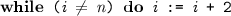), we have

Additional examples of termination analyses in the algebraic framework appear in  and .

### 5.2 The Instantiation of the Recipe

The recipe from Sect. 3.3 is instantiated for termination analysis as follows:

1. 1.

(Modeling). The concrete semantics is the set of non-terminating states, which is the greatest solution to a system of right-linear equations.

2. 2.

(Closed forms). The language of closed-forms is given by $$\omega$$-regular expressions; they can be computed by a variation of Tarjan’s algorithm .

3. 3.

(Interpretation). An $$\omega$$-regular expression can be interpreted as a state formula representing a set of possibly non-terminating states, while regular expressions are interpreted as transition formulas (Sect. 2). The soundness relation is over-approximate: we can prove that a program terminates by finding an unsatisfiable pre-condition, but the analysis cannot prove non-termination.

## 6 Recap

This section contains a few remarks about commonalities among the three kinds of problems and the techniques we have presented for applying algebraic program analysis to them. The paper has been structured around the three-part recipe for algebraic program analysis given in Sect. 3.3. Table 1 recaps how the recipe has been instantiated for the three kinds of problems considered.

Within this paper, all methods for computing closed-form solutions can be understood as some variation of Gaussian elimination, Algorithm 1 (in practice, they are variations of Tarjan’s path-expression algorithm). The essential difference between Sect. 2, Sect. 4, and Sect. 5 is the “loop-solving” step. Each requires the right-hand-side expression R to be in a particular form (left-linear, linear, right-linear), and each requires a different language of expressions in which to express closed forms (regular, tensored regular, $$\omega$$-regular). Table 2 shows the respective “loop-solving” steps for computing a closed form. Note that in Table 2, the letters $$a,b_i,c_i,z$$ range over expressions (which may involve variables other than X). For example, to apply the left-linear rule to the equation $$X = Xp + Xq + Yr + Z$$, we first re-arrange terms on the right-hand side as $$X(p+q) + (Yr+Z)$$ and then compute the “closed-form” $$(Yr+Z)(p+q)^*$$.

## 7 Related Work

Abstracting States Versus State Changes. Classically, invariant generation is conceived as the problem of over-approximating the reachable states of a program. Computing invariants involves solving a system of equations of the form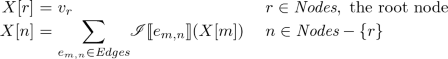(11)

for the unknowns X[n], $$n \in \textit{Nodes}$$, where $$v_r$$ represents the set of initial states and $$\mathscr {I}\llbracket {-}\rrbracket$$ provides an interpretation of each CFG edge as a state transformer. In a solution, X[n] holds a descriptor that represents a superset of the set of program states that can arise at program point n. Note that in Eq. (11), the function $$\mathscr {I}\llbracket {e_{m,n}}\rrbracket$$ on edge $$e_{m,n}$$ is applied to the value X[m] on node m.

Algebraic program analyses, in contrast, concern dynamics—state changes—rather than states. The reason is that algebraic analyses are compositional: states do not compose, but state changes do.

A first step towards abstracting state changes was taken by Graham & Wegman , who gave a method to solve dataflow equations via composition of the state transformers on CFG edges. That is, their basic primitives were (i) composition of functions, and (ii) union of functions. If we adopt this outlook and define $$r_1 \cdot r_2$$ to be $$r_2 \circ r_1$$, $$r_1 + r_2$$ to be the union of $$r_1$$ and $$r_2$$, and 1 to be the identity function, instead of Eq. (11), the goal would be to solve the following equation system: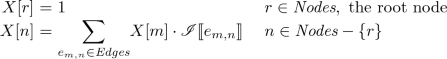(12)

where the unknowns X[n] are now function-valued. Note that the function $$\mathscr {I}\llbracket {e_{m,n}}\rrbracket$$ on edge $$e_{m,n}$$ is composed with the value X[m] on node m. From here—because one is working over function-valued quantities—it is now natural to formulate interprocedural program-analysis problems by means of equations over unknowns that denote procedure summaries, as was done by Cousot and Cousot  and Sharir and Pnueli .

“Interpret, Then Solve” Versus “Solve, Then Interpret.” The systems in Eqs. (11) and (12) are interpreted, in the sense that they are understood as semantic equations valued over a particular abstract domain, say D. Such a system $$E = \left\{ X_i = R_i \right\} _{i \in I}$$ can be solved by an iterative method: compute a sequence $$\sigma _0,\sigma _1,\dots \in \left\{ X_i \right\} _{i \in I} \rightarrow D$$ of assignments abstract domain values to variables

$$\begin{array}{rcl@{}l} \sigma _0(X_i) &{}\triangleq &{} 0 &{}\text {for all } i \in I\\ \sigma _{n+1}(X_i) &{}\triangleq &{} \mathscr {I}_{\sigma _{n}}\llbracket {R_i}\rrbracket &{} \text {for all }n \ge 0 \text { and all } i \in I \end{array}$$

Eventually this process converges—typically with the aid of widening to extrapolate to the limit—upon an assignment that over-approximates the least solution to E.

In algebraic program analysis, we think of a system of equations as an uninterpreted (syntactic) object. Equations are solved symbolically and then the solutions are interpreted in an algebraic structure to obtain an analysis result. The key step in this direction was made by Tarjan , who observed that once a solution to the path-expression problem was in hand, multiple dataflow-analysis problems could be solved merely by reinterpreting the alphabet symbols and operators of regular expressions in different algebras—i.e., “solve and then interpret.”

Whereas the iterative framework for program analysis has a “built-in” algorithm for analyzing loops and recursive behavior (by computing the limit of a sequence), the algebraic framework does not prescribe any particular method, and it is up to the analysis designer to devise one. This obligation places an additional burden on the analysis designer, but also provides flexibility: the analysis designer may analyze loops in ways that may (Example 6) or may not (Examples 5 and 4) resemble iterative fixpoint computation.

Iteration Operators and Loop Summarization. In the computer-aided-verification community, there is a body of literature on loop summarization (or “loop leaping”) and acceleration. Summarization aims to compute or approximate the behavior of (certain) loops, while acceleration aims to approximate the postimage of a set of states under a loop. These techniques have been incorporated into iterative abstract interpretation [28, 31], abstraction-refinement-based software model checking [19, 37], termination analysis [7, 20, 60], and resource bound analysis [10, 64]. The most closely related techniques to algebraic program analysis are those that build summaries for whole programs in “bottom-up” fashion. Such analyses have been formalized in various ways, including: recursion on the abstract syntax tree (AST) of a program , AST rewriting , and graph rewriting [47, 60]. Algebraic program analysis provides a unifying foundation for such analyses, in the same way that dataflow analysis  and (iterative) abstract interpretation  provide a unifying foundation for iterative program analyses.

There are several methods for loop summarization, based on finite-monoid affine transformations [11, 12, 29], difference-bound relations [15, 21], octagonal relations [13, 14, 45], integer vector addition systems , fragments of the theory of arrays . For the most part, these summarization methods are non-uniform in the sense that their input language differs from their output language (e.g.,  takes as input an octagonal relation and produces as output a Presburger formula). This non-uniformity is the essential barrier that must be overcome to use such techniques to implement the iteration operator of an algebraic program analysis (e.g., we can define an iteration operator by using optimization modulo theories  to extract the octagonal hull of a Presburger formula, then use  to compute a Presburger formula representing its transitive closure).

Elimination-Based Dataflow Analysis. Elimination-based dataflow analysis is a family of dataflow analyses that computes analysis results using methods that resemble Gaussian elimination [3, 33, 36] (see  for a survey). Early methods were specialized to reducible control flow graphs, but operated faster than general Gaussian elimination. Tarjan’s algorithm  is an elimination method with fast operation on reducible (and “nearly reducible”) control flow graphs, but is applicable to arbitrary graphs.

Weighted Graphs. There is a vast literature on solving path problems on weighted graphs where the weights are drawn from a semiring [1, 30, 50]. Path problems can also be solved on semiring-weighted pushdown systems, which has applications to interprocedural dataflow analysis . This work focuses on iterative techniques for solving path problems.

(Non-iterative) algorithms for path problems over algebraic structures with an explicit iteration operator were considered by Aho et al. , Backhouse & Carré , and Lehmann , and was implicit in previous work by Kleene , and McNaughton & Yamada . Tarjan connected this line of work with program analysis [58, 59].

## 8 Open Problems

We conclude with a list of challenges suggested by algebraic program analysis.

Scaling SMT-Based Algebraic Program Analysis. The bottom-up interpretation step of a closed-form expression is efficient, in that it operates in linear time and space in the size of the expression DAG in a model where each algebraic operation has unit cost. For logic-based interpretations, however, algebraic operations do not have unit cost: operators manipulate formulas, and the size of those formulas may grow as operators are applied. For example, the regular expression $$a^{2^n}$$ can be represented by an expression DAG with $$n+1$$ nodes, with the following shape:

If the letter a is interpreted as the transition formula $$x' = x + 1$$ and $$\cdot$$ as relational composition, then the transition-formula interpretation of $$a^{2^n}$$ has size $$O(2^n)$$. Scaling SMT-based algebraic program analysis to large programs requires techniques for generating succinct summaries, and/or efficient reasoning about compact formula representations involving $$\lambda$$-expressions.

Recursive Procedures. Section 4.2 shows how the algebraic approach can be applied to summarize linearly recursive procedures. But to compute summaries for generally recursive procedures, current-generation algebraic-program-analysis tools fall back on another non-algebraic scheme (such as hybrid iterative/algebraic, like Kleene or Newton iteration [40, 53], or the template-based approach of ). This raises the question: is there a practical algebraic method for analyzing general recursion? The essential challenge is in devising a language of “closed forms” that (1) can represent arbitrary context-free languages, and (2) is amenable to an effective interpretation in logic.

Beyond Numerical Domains. To date, all algebraic program analyses have been numerical in nature—they abstract away aspects of program behavior that cannot be captured by integer variables. It remains to be seen whether the algebraic approach can yield practical analyses for reasoning about features like strings, arrays, and the heap. Reasoning about memory manipulation is particularly challenging in a compositional setting, since we cannot rely on the context of a program fragment to resolve aliasing relationships. One possible avenue is to incorporate abductive reasoning to make educated guesses about the shape of memory, as in .

Property Refutation. Algebraic program analysis is typically conceived as a method for generating over-approximate summaries. The nature of over-approximation is that the summaries can be used to verify that a program does satisfy a property of interest, but not prove that it doesn’t. An interesting direction for future work is to devise methods by which algebraic program analyses can refute properties, perhaps based on bounded model checking , under-approximate loop summarization , or symbolic execution .